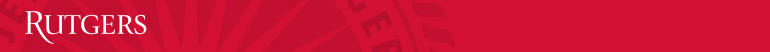﻿ General PhysicsMath & Science Learning Center﻿ sch
• Group Problems: are graded out of 5 points; The short quiz is graded out of 2 points. Three more points are earned based on the completed group worksheets. Partial credit for each question may be 0.25, 0.50 or 0.75.
• The following grades: 0late 0abs (absent), 0ns (not submitted) are assigned respectively to the following cases: Lateness for classes, absences and not submitted assignments.
• Quiz and Lab: are graded out of 5 points; The  Quiz is graded out of 3 points (one point per question). Partial credit for each question may be 0.25, 0.50 or 0.75. Two more points are earned during class based on work habit and performance.
• The following grades: 0late 0abs (absent), 0ns (not submitted) are assigned respectively for the following cases: Lateness for classes, absences and not turned in homework.
• Online Homework: are automatically graded out of 10 points, (one point per question). There is no partial credit for online homework.
• Exams: There are two midterm exams, each consisting of 15 multiple choice questions. The final exam has 30 multiple choice questions. There is no partial credit for exams.
 Required Assignments Percentage Exam 1 15 Exam 2 15 Final 30 Group Problems 15 Lab & Quizzes 15 Online Homework 10